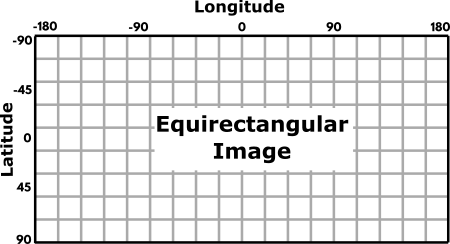# Class EquirectangularTools_F32

java.lang.Object
boofcv.alg.distort.spherical.EquirectangularTools_F32

```@Generated("boofcv.alg.distort.spherical.EquirectangularTools_F64")
public class EquirectangularTools_F32
extends Object```
Contains common operations for handling coordinates in an equirectangular image. On most globes, a positive latitude corresponds to the north pole, or up, and negative towards the south pole. Images have 0 on the top and increase downwards. To compensate for this the y-axis can be flipped. This is indicated by functions with FV (flip vertical) on the end of their name. Coordinate System:
• longitude is along the x-axis and goes from -pi to pi
• latitude is along the y-axis and goes from -pi/2 to pi/2
• image center (width/2, (height-1)/2.0ff) or (lat=0, lon=0) corresponds to a unit sphere of (1,0,0)
• unit sphere of (0,0,1) is pixel (width/2,0) and (0,0,-1) is (width/2,height-1)
• unit sphere of (0,1,0) is pixel (3*width/4,(height-1)/2) and (0,0,-1) is (width/4,(height-1)/2)
Coordinate System with y-flipped:
• longitude is along the x-axis and goes from -pi to pi
• latitude is along the y-axis and goes from pi/2 to -pi/2
• image center (width/2, height/2) or (lat=0, lon=0) corresponds to a unit sphere of (1,0,0)
• unit sphere of (0,0,1) is pixel (width/2,height-1) and (0,0,-1) is (width/2,0)
• unit sphere of (0,1,0) is pixel (3*width/4,(height-1)/2) and (0,0,-1) is (width/4,(height-1)/2)

Latitude and Longitude shown on a sphere in equirectangular format. Note that y is NOT flipped and angles are shown in degrees not radians git push.• ## Constructor Summary

Constructors
Constructor Description
`EquirectangularTools_F32()`
• ## Method Summary

Modifier and Type Method Description
`void` ```configure​(int width, int height)```
Specifies the image and which latitude/longtiude will comprise the center axises
`void` ```equiToLatLon​(float x, float y, GeoLL_F32 geo)```
Converts the equirectangular coordinate into a latitude and longitude
`void` ```equiToLatLonFV​(float x, float y, GeoLL_F32 geo)```
Converts the equirectangular coordinate into a latitude and longitude.
`void` ```equiToNorm​(float x, float y, Point3D_F32 norm)```
Converts equirectangular into normalized pointing vector
`void` ```equiToNormFV​(float x, float y, Point3D_F32 norm)```
`int` `getHeight()`
`int` `getWidth()`
`void` ```latlonToEqui​(float lat, float lon, Point2D_F32 rect)```
Convert from latitude-longitude coordinates into equirectangular coordinates
`void` ```latlonToEquiFV​(float lat, float lon, Point2D_F32 rect)```
Convert from latitude-longitude coordinates into equirectangular coordinates.
`void` ```normToEqui​(float nx, float ny, float nz, Point2D_F32 rect)```
`void` ```normToEquiFV​(float nx, float ny, float nz, Point2D_F32 rect)```

### Methods inherited from class java.lang.Object

`clone, equals, finalize, getClass, hashCode, notify, notifyAll, toString, wait, wait, wait`
• ## Constructor Details

• ### EquirectangularTools_F32

public EquirectangularTools_F32()
• ## Method Details

• ### configure

public void configure​(int width, int height)
Specifies the image and which latitude/longtiude will comprise the center axises
Parameters:
`width` - Image width
`height` - Image height
• ### equiToNorm

public void equiToNorm​(float x, float y, Point3D_F32 norm)
Converts equirectangular into normalized pointing vector
Parameters:
`x` - pixel coordinate in equirectangular image
`y` - pixel coordinate in equirectangular image
`norm` - Normalized pointing vector
• ### normToEqui

public void normToEqui​(float nx, float ny, float nz, Point2D_F32 rect)
• ### equiToNormFV

public void equiToNormFV​(float x, float y, Point3D_F32 norm)
• ### normToEquiFV

public void normToEquiFV​(float nx, float ny, float nz, Point2D_F32 rect)
• ### equiToLatLon

public void equiToLatLon​(float x, float y, GeoLL_F32 geo)
Converts the equirectangular coordinate into a latitude and longitude
Parameters:
`x` - pixel coordinate in equirectangular image
`y` - pixel coordinate in equirectangular image
`geo` - (output)
• ### equiToLatLonFV

public void equiToLatLonFV​(float x, float y, GeoLL_F32 geo)

Converts the equirectangular coordinate into a latitude and longitude. Vertical equirectangular axis has been flipped

y' = height - y - 1
Parameters:
`x` - pixel coordinate in equirectangular image
`y` - pixel coordinate in equirectangular image
`geo` - (output)
• ### latlonToEqui

public void latlonToEqui​(float lat, float lon, Point2D_F32 rect)
Convert from latitude-longitude coordinates into equirectangular coordinates
Parameters:
`lat` - Latitude
`lon` - Longitude
`rect` - (Output) equirectangular coordinate
• ### latlonToEquiFV

public void latlonToEquiFV​(float lat, float lon, Point2D_F32 rect)
Convert from latitude-longitude coordinates into equirectangular coordinates. Vertical equirectangular axis has been flipped
Parameters:
`lat` - Latitude
`lon` - Longitude
`rect` - (Output) equirectangular coordinate
• ### getWidth

public int getWidth()
• ### getHeight

public int getHeight()